# forecast

Forecast univariate autoregressive integrated moving average (ARIMA) model responses or conditional variances

## Syntax

``````[Y,YMSE] = forecast(Mdl,numperiods,Y0)``````
``[Y,YMSE] = forecast(Mdl,numperiods,Y0,Name,Value)``
``````[Y,YMSE,V] = forecast(___)``````

## Description

example

``````[Y,YMSE] = forecast(Mdl,numperiods,Y0)``` returns `numperiods` consecutive forecasted responses `Y` and corresponding mean square errors (MSE) `YMSE` of the fully specified, univariate ARIMA model `Mdl`. The presample response data `Y0` initializes the model to generate forecasts.```

example

``[Y,YMSE] = forecast(Mdl,numperiods,Y0,Name,Value)` uses additional options specified by one or more name-value arguments. For example, for a model with a regression component (that is, an ARIMAX model), `'X0',X0,'XF',XF` specifies the presample and forecasted predictor data `X0` and `XF`, respectively.`

example

``````[Y,YMSE,V] = forecast(___)``` also forecasts `numperiods` conditional variances `V` of a composite conditional mean and variance model (for example, an ARIMA and GARCH composite model) using any of the input argument combinations in the previous syntaxes.```

## Examples

collapse all

Forecast the conditional mean response of simulated data over a 30-period horizon.

Simulate 130 observations from a multiplicative seasonal moving average (MA) model with known parameter values.

```Mdl = arima('MA',{0.5,-0.3},'SMA',0.4,'SMALags',12,... 'Constant',0.04,'Variance',0.2); rng(200); Y = simulate(Mdl,130);```

Fit a seasonal MA model to the first 100 observations, and reserve the remaining 30 observations to evaluate forecast performance.

```MdlTemplate = arima('MALags',1:2,'SMALags',12); EstMdl = estimate(MdlTemplate,Y(1:100));```
``` ARIMA(0,0,2) Model with Seasonal MA(12) (Gaussian Distribution): Value StandardError TStatistic PValue ________ _____________ __________ __________ Constant 0.20403 0.069064 2.9542 0.0031344 MA{1} 0.50212 0.097298 5.1606 2.4619e-07 MA{2} -0.20174 0.10447 -1.9312 0.053464 SMA{12} 0.27028 0.10907 2.478 0.013211 Variance 0.18681 0.032732 5.7073 1.148e-08 ```

`EstMdl` is a new `arima` model that contains estimated parameters (that is, a fully specified model).

Forecast the fitted model into a 30-period horizon. Specify the estimation period data as a presample.

```[YF,YMSE] = forecast(EstMdl,30,Y(1:100)); YF(15)```
```ans = 0.2040 ```
`YMSE(15)`
```ans = 0.2592 ```

`YF` is a 30-by-1 vector of forecasted responses, and `YMSE` is a 30-by-1 vector of corresponding MSEs. The 15-period-ahead forecast is 0.2040 and its MSE is 0.2592.

Visually compare the forecasts to the holdout data.

```figure h1 = plot(Y,'Color',[.7,.7,.7]); hold on h2 = plot(101:130,YF,'b','LineWidth',2); h3 = plot(101:130,YF + 1.96*sqrt(YMSE),'r:',... 'LineWidth',2); plot(101:130,YF - 1.96*sqrt(YMSE),'r:','LineWidth',2); legend([h1 h2 h3],'Observed','Forecast',... '95% Confidence Interval','Location','NorthWest'); title(['30-Period Forecasts and Approximate 95% '... 'Confidence Intervals']) hold off```Forecast the daily NASDAQ Composite Index over a 500-day horizon.

Load the NASDAQ data set, and extract the first 1500 observations.

```load Data_EquityIdx nasdaq = DataTable.NASDAQ(1:1500);```

Fit an ARIMA(1,1,1) model to the data.

```nasdaqModel = arima(1,1,1); nasdaqFit = estimate(nasdaqModel,nasdaq);```
``` ARIMA(1,1,1) Model (Gaussian Distribution): Value StandardError TStatistic PValue _________ _____________ __________ __________ Constant 0.43031 0.18555 2.3191 0.020392 AR{1} -0.074391 0.081985 -0.90737 0.36421 MA{1} 0.31126 0.077266 4.0284 5.6158e-05 Variance 27.826 0.63625 43.735 0 ```

Forecast the Composite Index for 500 days using the fitted model. Use the observed data as presample data.

`[Y,YMSE] = forecast(nasdaqFit,500,nasdaq);`

Plot the forecasts and 95% forecast intervals.

```lower = Y - 1.96*sqrt(YMSE); upper = Y + 1.96*sqrt(YMSE); figure plot(nasdaq,'Color',[.7,.7,.7]); hold on h1 = plot(1501:2000,lower,'r:','LineWidth',2); plot(1501:2000,upper,'r:','LineWidth',2) h2 = plot(1501:2000,Y,'k','LineWidth',2); legend([h1 h2],'95% Interval','Forecast',... 'Location','NorthWest') title('NASDAQ Composite Index Forecast') hold off```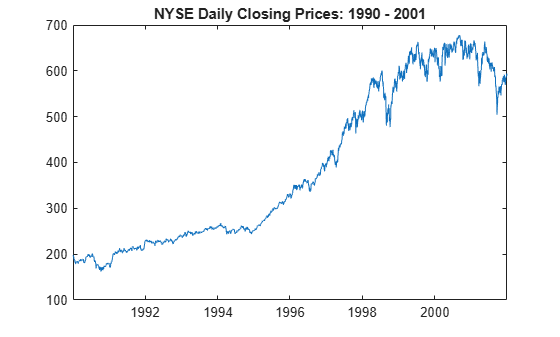The process is nonstationary, so the width of each forecast interval grows with time.

Forecast the following known autoregressive model with one lag and an exogenous predictor (ARX(1)) model into a 10-period forecast horizon:

`${y}_{t}=1+0.3{y}_{t-1}+2{x}_{t}+{\epsilon }_{t},$`

where ${\epsilon }_{\mathit{t}}$ is a standard Gaussian random variable, and ${\mathit{x}}_{\mathit{t}}$ is an exogenous Gaussian random variable with a mean of 1 and a standard deviation of 0.5.

Create an `arima` model object that represents the ARX(1) model.

`Mdl = arima('Constant',1,'AR',0.3,'Beta',2,'Variance',1);`

To forecast responses from the ARX(1) model, the `forecast` function requires:

• One presample response ${\mathit{y}}_{0}$ to initialize the autoregressive term

• Future exogenous data to include the effects of the exogenous variable on the forecasted responses

Set the presample response to the unconditional mean of the stationary process:

`$E\left({y}_{t}\right)=\frac{1+2\left(1\right)}{1-0.3}.$`

For the future exogenous data, draw 10 values from the distribution of the exogenous variable.

```rng(1); y0 = (1 + 2)/(1 - 0.3); xf = 1 + 0.5*randn(10,1);```

Forecast the ARX(1) model into a 10-period forecast horizon. Specify the presample response and future exogenous data.

```fh = 10; yf = forecast(Mdl,fh,y0,'XF',xf)```
```yf = 10×1 3.6367 5.2722 3.8232 3.0373 3.0657 3.3470 3.4454 4.2120 4.0667 4.8065 ```

`yf(3)` = `3.8232` is the 3-period-ahead forecast of the ARX(1) model.

Forecast multiple response paths from a known SAR$\left(1,0,0\right){\left(1,1,0\right)}_{4}$ model by specifying multiple presample response paths.

Create an `arima` model object that represents this quarterly SAR$\left(1,0,0\right){\left(1,1,0\right)}_{4}$ model:

`$\left(1-0.5L\right)\left(1-0.2{L}^{4}\right)\left(1-{L}^{4}\right){y}_{t}=1+{\epsilon }_{t},$`

where ${\epsilon }_{\mathit{t}}$ is a standard Gaussian random variable.

```Mdl = arima('Constant',1,'AR',0.5,'Variance',1,... 'Seasonality',4,'SARLags',4,'SAR',0.2)```
```Mdl = arima with properties: Description: "ARIMA(1,0,0) Model Seasonally Integrated with Seasonal AR(4) (Gaussian Distribution)" Distribution: Name = "Gaussian" P: 9 D: 0 Q: 0 Constant: 1 AR: {0.5} at lag  SAR: {0.2} at lag  MA: {} SMA: {} Seasonality: 4 Beta: [1×0] Variance: 1 ```

Because `Mdl` contains autoregressive dynamic terms, `forecast` requires the previous `Mdl.P` responses to generate a $\mathit{t}$-period-ahead forecast from the model. Therefore, the presample must contain at least nine values.

Generate a random 9-by-10 matrix representing 10 presample paths of length 9.

```rng(1); numpaths = 10; Y0 = rand(Mdl.P,numpaths);```

Forecast 10 paths from the SAR model into a 12-quarter forecast horizon. Specify the presample observation paths `Y0`.

```fh = 12; YF = forecast(Mdl,fh,Y0);```

`YF` is a 12-by-10 matrix of independent forecasted paths. `YF(j,k)` is the `j`-period-ahead forecast of path `k`. Path `YF(:,k)` represents the continuation of the presample path `Y0(:,k)`.

Plot the presample and forecasts.

```Y = [Y0;... YF]; figure; plot(Y); hold on h = gca; px = [6.5 h.XLim([2 2]) 6.5]; py = h.YLim([1 1 2 2]); hp = patch(px,py,[0.9 0.9 0.9]); uistack(hp,"bottom"); axis tight legend("Forecast period") xlabel('Time (quarters)') ylabel('Response paths')```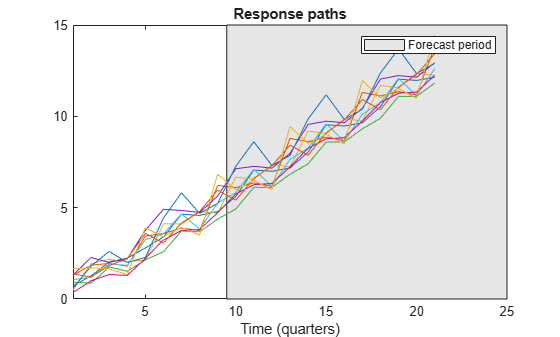Consider the following AR(1) conditional mean model with a GARCH(1,1) conditional variance model for the daily NASDAQ rate series (as a percent) from January 2, 1990 through December 31, 2001.

`$\begin{array}{l}{y}_{t}=0.073+0.138{y}_{t-1}+{\epsilon }_{t}\\ {\sigma }_{t}^{2}=0.022+0.873{\sigma }_{t-1}^{2}+0.119{\epsilon }_{t-1},\end{array}$`

where ${\epsilon }_{\mathit{t}}$ is a series of independent random Gaussian variables with a mean of 0.

Create the model.

```CondVarMdl = garch('Constant',0.022,'GARCH',0.873,'ARCH',0.119); Mdl = arima('Constant',0.073,'AR',0.138,'Variance',CondVarMdl);```

Load the equity index data set. Convert the table to a timetable, and convert the NASDAQ price series to a return series. Because the return series has one less observation than the price series, prepad the return series to synchronize it with variables in the timetable.

```load Data_EquityIdx dates = datetime(dates,'ConvertFrom','datenum','Locale','en_US'); TT = table2timetable(DataTable,'RowTimes',dates); T = size(TT,1); y0 = 100*price2ret(DataTable.NASDAQ); [e0,v0] = infer(Mdl,y0); n = numel(y0); TT{:,["NASDAQRet" "Residuals" "CondVar"]} = [nan(T-n,3); y0 e0 v0];```

Forecast the model over a 25-day horizon. Supply the entire data set as a presample (`forecast` uses only the latest required observations to initialize the conditional mean and variance models). Return forecasted responses and conditional variances.

```fh = 25; fhdates = TT.Time(end) + caldays(0:fh); % Forecast horizon dates [y,~,v] = forecast(Mdl,fh,TT.NASDAQRet);```

Plot the forecasted responses and conditional variances with the observed series from August 2001.

```pdates = TT.Time > datetime(2001,8,1); plot(TT.Time(pdates),TT.NASDAQRet(pdates)) hold on plot(fhdates,[TT.NASDAQRet(end); y]) hold off```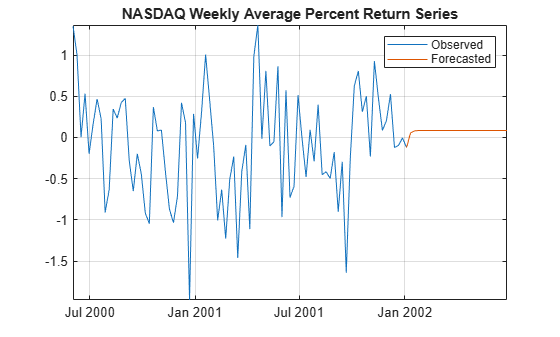```plot(TT.Time(pdates),TT.CondVar(pdates)) hold on plot(fhdates,[TT.CondVar(end); v]); hold off```## Input Arguments

collapse all

Fully specified ARIMA model, specified as an `arima` model object created by `arima` or `estimate`.

The properties of `Mdl` cannot contain `NaN` values.

Forecast horizon, or the number of time points in the forecast period, specified as a positive integer.

Data Types: `double`

Presample response data paths used to initialize the model for forecasting, specified as a numeric column vector with length `numpreobs` or a `numpreobs`-by-`numpaths` numeric matrix.

Rows of `Y0` correspond to periods in the presample, and the last row contains the latest presample response. `numpreobs` is the number of specified presample responses, which must be at least `Mdl.P`. If `numpreobs` exceeds `Mdl.P`, the `forecast` function uses only the latest `Mdl.P` rows. For more details, see Time Base Partitions for Forecasting.

Columns of `Y0` correspond to separate, independent presample paths.

• If `Y0` is a column vector, `forecast` applies it to each forecasted path. In this case, all forecast paths `Y` derive from the same initial conditions.

• If `Y0` is a matrix, it must have `numpaths` columns, where `numpaths` is the maximum among the second dimensions of the specified presample observation arrays `Y0`, `E0`, and `V0`.

Data Types: `double`

### Name-Value Arguments

Specify optional pairs of arguments as `Name1=Value1,...,NameN=ValueN`, where `Name` is the argument name and `Value` is the corresponding value. Name-value arguments must appear after other arguments, but the order of the pairs does not matter.

Before R2021a, use commas to separate each name and value, and enclose `Name` in quotes.

Example: `'X0',X0,'XF',XF` specifies the presample and forecasted predictor data `X0` and `XF`, respectively.

Presample innovations used to initialize either the moving average (MA) component of the ARIMA model or the conditional variance model, specified as a numeric column vector or a numeric matrix with `numpaths` columns. `forecast` assumes that the presample innovations have a mean of 0.

Rows of `E0` correspond to periods in the presample, and the last row contains the latest presample innovation. `E0` must have at least `Mdl.Q` rows to initialize the MA component. If `Mdl.Variance` is a conditional variance model (for example, a `garch` model object), `E0` might require more than `Mdl.Q` rows. If the number of rows exceeds the minimum number required to forecast `Mdl`, the `forecast` function uses only the latest required rows.

Columns of `E0` correspond to separate, independent presample paths.

• If `E0` is a column vector, `forecast` applies it to each forecasted path. In this case, the MA component and conditional variance model of all forecast paths `Y` derive from the same initial innovations.

• If `E0` is a matrix, it must have `numpaths` columns.

• By default, if `numpreobs``Mdl.P` + `Mdl.Q`, `forecast` infers any necessary presample innovations by passing the model `Mdl` and presample data to the `infer` function. For details on the default for models containing a regression component, see `X0` and `XF`.

• By default, if `numpreobs` < `Mdl.P` + `Mdl.Q`, `forecast` sets all necessary presample innovations to `0`.

Data Types: `double`

Presample conditional variances used to initialize the conditional variance model, specified as a positive numeric column vector or a positive numeric matrix with `numpaths` columns. If the model variance `Mdl.Variance` is constant, `forecast` ignores `V0`.

Rows of `V0` correspond to periods in the presample, and the last row contains the latest presample conditional variance. If `Mdl.Variance` is a conditional variance model (for example, a `garch` model object), `E0` might require more than `Mdl.Q` rows to initialize `Mdl` for forecasting. If the number of rows exceeds the minimum number required to forecast `Mdl`, the `forecast` function uses only the latest required presample conditional variances.

Columns of `V0` correspond to separate, independent presample paths.

• If `V0` is a column vector, `forecast` applies it to each forecasted path. In this case, the conditional variance model of all forecast paths `Y` derive from the same initial conditional variances.

• If `V0` is a matrix, it must have `numpaths` columns.

By default:

• If you specify enough presample innovations `E0` to initialize the conditional variance model `Mdl.Variance`, `forecast` infers any necessary presample conditional variances by passing the conditional variance model and `E0` to the `infer` function.

• If you do not specify `E0`, but you specify enough presample responses `Y0` to infer enough presample innovations, then `forecast` infers any necessary presample conditional variances from the inferred presample innovations.

• If you do not specify enough presample data, `forecast` sets all necessary presample conditional variances to the unconditional variance of the variance process.

Data Types: `double`

Presample predictor data used to infer the presample innovations `E0`, specified as a numeric matrix with `numpreds` columns.

Rows of `X0` correspond to periods in the presample, and the last row contains the latest set of presample predictor observations. Columns of `X0` represent separate time series variables, and they correspond to the columns of `XF`.

If you do not specify `E0`, `X0` must have at least `numpreobs``Mdl.P` rows so that `forecast` can infer presample innovations. If the number of rows exceeds the minimum number required to infer presample innovations, `forecast` uses only the latest required presample predictor observations. A best practice is to set `X0` to the same predictor data matrix used in the estimation, simulation, or inference of `Mdl`. This setting ensures the correct estimation of the presample innovations `E0`.

If you specify `E0`, then `forecast` ignores `X0`.

If you specify `X0` but you do not specify forecasted predictor data `XF`, then `forecast` issues an error.

By default, `forecast` drops the regression component from the model when it infers presample innovations, regardless of the value of the regression coefficient `Mdl.Beta`.

Data Types: `double`

Forecasted (or future) predictor data, specified as a numeric matrix with `numpreds` columns. `XF` represents the evolution of specified presample predictor data `X0` forecasted into the future (the forecast period).

Rows of `XF` correspond to time points in the future; `XF(t,:)` contains the `t`-period-ahead predictor forecasts. `XF` must have at least `numperiods` rows. If the number of rows exceeds `numperiods`, `forecast` uses only the first `numperiods` forecasts. For more details, see Time Base Partitions for Forecasting.

Columns of `XF` are separate time series variables, and they correspond to the columns of `X0`.

By default, the `forecast` function generates forecasts from `Mdl` without a regression component, regardless of the value of the regression coefficient `Mdl.Beta`.

Note

`forecast` assumes that you synchronize all specified presample data sets so that the latest observation of each presample series occurs simultaneously. Similarly, `forecast` assumes that the first observation in the forecasted predictor data `XF` occurs in the time point immediately after the last observation in the presample predictor data `X0`.

## Output Arguments

collapse all

Minimum mean square error (MMSE) forecasts of the conditional mean of the response series, returned as a length `numperiods` column vector or a `numperiods`-by-`numpaths` numeric matrix. `Y` represents a continuation of `Y0` (`Y(1,:)` occurs in the time point immediately after `Y0(end,:)`).

`Y(t,:)` contains the `t`-period-ahead forecasts, or the conditional mean forecast of all paths for time point `t` in the forecast period.

`forecast` determines `numpaths` from the number of columns in the presample data sets `Y0`, `E0`, and `V0`. For details, see Algorithms. If each presample data set has one column, then `Y` is a column vector.

Data Types: `double`

MSE of the forecasted responses `Y` (forecast error variances), returned as a length `numperiods` column vector or a `numperiods`-by-`numpaths` numeric matrix.

`YMSE(t,:)` contains the forecast error variances of all paths for time point `t` in the forecast period.

`forecast` determines `numpaths` from the number of columns in the presample data sets `Y0`, `E0`, and `V0`. For details, see Algorithms. If you do not specify any presample data sets, or if each data set is a column vector, then `YMSE` is a column vector.

The square roots of `YMSE` are the standard errors of the forecasts `Y`.

Data Types: `double`

MMSE forecasts of the conditional variances of future model innovations, returned as a length `numperiods` numeric column vector or a `numperiods`-by-`numpaths` numeric matrix. `V` has `numperiods` rows and `numpaths` columns.

`forecast` sets the number of columns of `V` (`numPaths`) to the largest number of columns in the presample arrays `Y0`, `E0`, and `V0`. If you do not specify `Y0`, `E0`, and `V0`, then `V` is a `numPeriods` column vector.

In all cases, row `j` contains the conditional variance forecasts of period `j`.

Data Types: `double`

collapse all

### Time Base Partitions for Forecasting

Time base partitions for forecasting are two disjoint, contiguous intervals of the time base; each interval contains time series data for forecasting a dynamic model. The forecast period (forecast horizon) is a `numperiods` length partition at the end of the time base during which the `forecast` function generates the forecasts `Y` from the dynamic model `Mdl`. The presample period is the entire partition occurring before the forecast period. The `forecast` function can require observed responses `Y0`, innovations `E0`, or conditional variances `V0` in the presample period to initialize the dynamic model for forecasting. The model structure determines the types and amounts of required presample observations.

A common practice is to fit a dynamic model to a portion of the data set, and then validate the predictability of the model by comparing its forecasts to observed responses. During forecasting, the presample period contains the data to which the model is fit, and the forecast period contains the holdout sample for validation. Suppose that yt is an observed response series; x1,t, x2,t, and x3,t are observed exogenous series; and time t = 1,…,T. Consider forecasting responses from a dynamic model of yt containing a regression component with `numperiods` = K periods. Suppose that the dynamic model is fit to the data in the interval [1,TK] (for more details, see `estimate`). This figure shows the time base partitions for forecasting.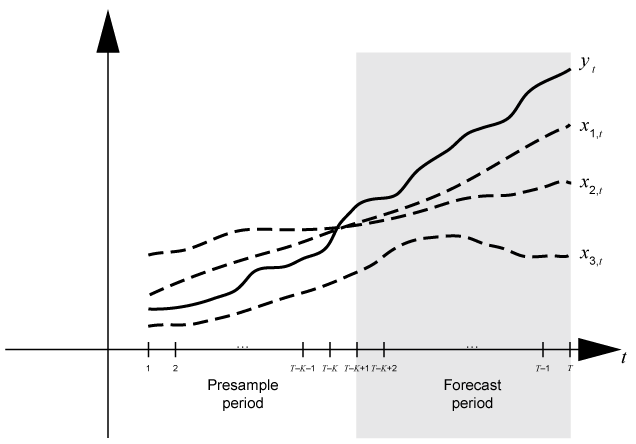For example, to generate the forecasts `Y` from an ARX(2) model, `forecast` requires:

• Presample responses `Y0` = ${\left[\begin{array}{cc}{y}_{T-K-1}& {y}_{T-K}\end{array}\right]}^{\prime }$ to initialize the model. The 1-period-ahead forecast requires both observations, whereas the 2-periods-ahead forecast requires yTK and the 1-period-ahead forecast `Y(1)`. The `forecast` function generates all other forecasts by substituting previous forecasts for lagged responses in the model.

• Future exogenous data `XF` = $\left[\begin{array}{ccc}{x}_{1,\left(T-K+1\right):T}& {x}_{2,\left(T-K+1\right):T}& {x}_{3,\left(T-K+1\right):T}\end{array}\right]$ for the model regression component. Without specified future exogenous data, the `forecast` function ignores the model regression component, which can yield unrealistic forecasts.

Dynamic models containing either a moving average component or a conditional variance model can require presample innovations or conditional variances. Given enough presample responses, `forecast` infers the required presample innovations and conditional variances. If such a model also contains a regression component, then `forecast` must have enough presample responses and exogenous data to infer the required presample innovations and conditional variances. This figure shows the arrays of required observations for this case, with corresponding input and output arguments.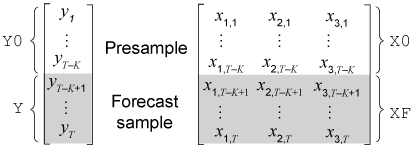## Algorithms

• The `forecast` function sets the number of sample paths (`numpaths`) to the maximum number of columns among the presample data sets `E0`, `V0`, and `Y0`. All presample data sets must have either one column or `numpaths` > 1 columns. Otherwise, `forecast` issues an error. For example, if you supply `Y0` and `E0`, and `Y0` has five columns representing five paths, then `E0` can each have one column or five columns. If `E0` has one column, `forecast` applies `E0` to each path.

• `NaN` values in presample and future data sets indicate missing data. `forecast` removes missing data from the presample data sets following this procedure:

1. `forecast` horizontally concatenates the specified presample data sets `Y0`, `E0`, `V0`, and `X0` so that the latest observations occur simultaneously. The result can be a jagged array because the presample data sets can have a different number of rows. In this case, `forecast` prepads variables with an appropriate number of zeros to form a matrix.

2. `forecast` applies list-wise deletion to the combined presample matrix by removing all rows containing at least one `NaN`.

3. `forecast` extracts the processed presample data sets from the result of step 2, and removes all prepadded zeros.

`forecast` applies a similar procedure to the forecasted predictor data `XF`. After `forecast` applies list-wise deletion to `XF`, the result must have at least `numperiods` rows. Otherwise, `forecast` issues an error.

List-wise deletion reduces the sample size and can create irregular time series.

• When `forecast` estimates the MSEs `YMSE` of the conditional mean forecasts `Y`, the function treats the specified predictor data sets `X0` and `XF` as exogenous, nonstochastic, and statistically independent of the model innovations. Therefore, `YMSE` reflects only the variance associated with the ARIMA component of the input model `Mdl`.

 Baillie, Richard T., and Tim Bollerslev. “Prediction in Dynamic Models with Time-Dependent Conditional Variances.” Journal of Econometrics 52, (April 1992): 91–113. https://doi.org/10.1016/0304-4076(92)90066-Z.

 Bollerslev, Tim. “Generalized Autoregressive Conditional Heteroskedasticity.” Journal of Econometrics 31 (April 1986): 307–27. https://doi.org/10.1016/0304-4076(86)90063-1.

 Bollerslev, Tim. “A Conditionally Heteroskedastic Time Series Model for Speculative Prices and Rates of Return.” The Review of Economics and Statistics 69 (August 1987): 542–47. https://doi.org/10.2307/1925546.

 Box, George E. P., Gwilym M. Jenkins, and Gregory C. Reinsel. Time Series Analysis: Forecasting and Control. 3rd ed. Englewood Cliffs, NJ: Prentice Hall, 1994.

 Enders, Walter. Applied Econometric Time Series. Hoboken, NJ: John Wiley & Sons, Inc., 1995.

 Engle, Robert. F. “Autoregressive Conditional Heteroscedasticity with Estimates of the Variance of United Kingdom Inflation.” Econometrica 50 (July 1982): 987–1007. https://doi.org/10.2307/1912773.

 Hamilton, James D. Time Series Analysis. Princeton, NJ: Princeton University Press, 1994.